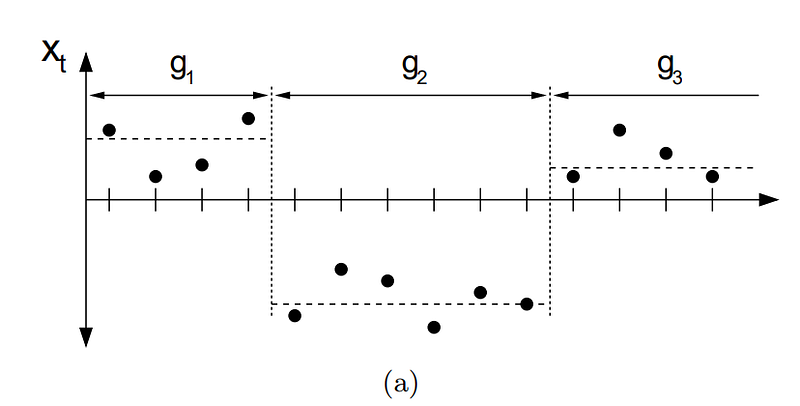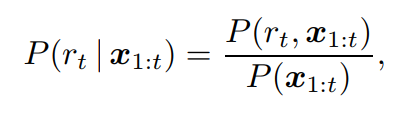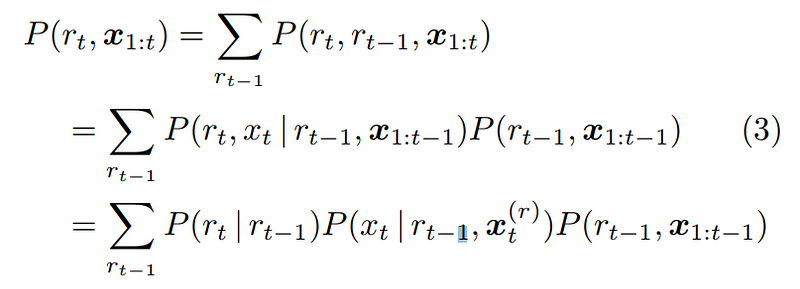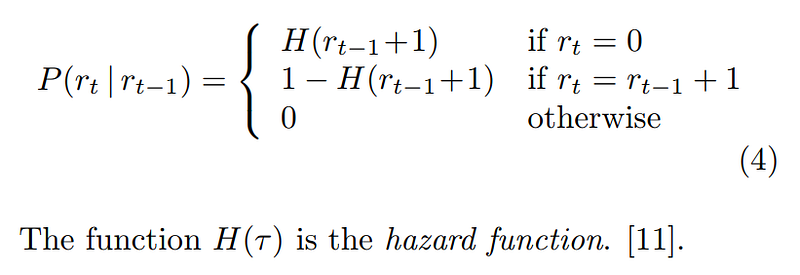Search
• Madalina Ciortan

# Bayesian multiple change point detection

Recursive estimation of change pointsChange points are abrupt changes in a sequence of observations which split the observed data in one (single change point) or more (multiple change point) segments characterized by different data distribution, as depicted in the figure below. Being able to detect change points provides many valuable applications when working with time series (for instance finance, robotics or data science in general).This post is based on Ryan Prescott Adam’s paper and is a showcase of the implementation proposed here.

We assume that the observed values x1, x2, … xN can be divided into T non-overlapping segments, each characterized by some probability distribution. The implementation chooses Student-t as it is a heavy-tailed distribution and provides a better fit for data with atypical observations than normal distributions for instance.

The goal is to estimate the posterior distribution of observations since the last change point, given observed data. If we denote by rt the length of the current run at time t, we would like to find the posterior distribution:which is shown to depend recursively on the prior over rt given rt-1(first term below) and the predictive distribution over the newly observed data since the last change point (second term).The first term is equal to a hazard function when a change point occurs and rt =0 and 1- hazard function when the run length continues:This notebook shows an example of using in practice the above implementation.

### Other approaches

Another approach based on a non-parametric estimation of both the number of change points and there position has been recently proposed by Matteson, David S., and Nicholas A. James. “A nonparametric approach for multiple change point analysis of multivariate data.” Journal of the American Statistical Association 109.505 (2014): 334–345.

See All